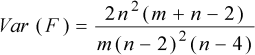# Snedecor LawThe Snedecor law is parameterized by two non-dimensional numbers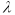and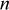.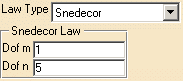Let's take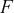a random variable following the Snedecor law of parametersand, thencan be expressed in terms of two random variables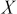,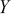following Pearson law respectively of parameter,as: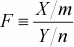is distributed according to the following density of probability, where: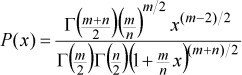With mean: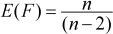With variance: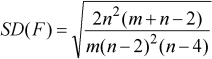With standard deviation: